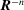International
Tables for
Crystallography
Volume B
Reciprocal space
Edited by U. Shmueli

International Tables for Crystallography (2006). Vol. B, ch. 3.4, pp. 385-397   | 1 | 2 |
https://doi.org/10.1107/97809553602060000562

## Chapter 3.4. Accelerated convergence treatment of R−n lattice sums

D. E. Williamsa

aDepartment of Chemistry, University of Louisville, Louisville, Kentucky 40292, USA

### References

Arfken, G. (1970). Mathematical methods for physicists, 2nd ed. New York: Academic Press.Google Scholar
Bertaut, E. F. (1952). L'énergie électrostatique de réseaux ioniques. J. Phys. (Paris), 13, 499–505.Google Scholar
Bertaut, E. F. (1978). The equivalent charge concept and its application to the electrostatic energy of charges and multipoles. J. Phys. (Paris), 39, 1331–1348.Google Scholar
Busing, W. R. (1981). WMIN, a computer program to model molecules and crystals in terms of potential energy functions. Oak Ridge National Laboratory Report ORNL-5747. Oak Ridge, Tennessee 37830, USA.Google Scholar
Cummins, P. G., Dunmur, D. A., Munn, R. W. & Newham, R. J. (1976). Applications of the Ewald method. I. Calculation of multipole lattice sums. Acta Cryst. A32, 847–853.Google Scholar
Davis, P. J. (1972). Gamma function and related functions. Handbook of mathematical functions with formulas, graphs, and mathematical tables, edited by M. Abramowitz & I. A. Stegun, pp. 260–262. London, New York: John Wiley. [Reprint, with corrections of 1964 Natl Bur. Stand. publication.]Google Scholar
DeWette, F. W. & Schacher, G. E. (1964). Internal field in general dipole lattices. Phys. Rev. 137, A78–A91.Google Scholar
Evjen, H. M. (1932). The stability of certain heteropolar crystals. Phys. Rev. 39, 675–694.Google Scholar
Ewald, P. P. (1921). Die Berechnung optischer und elektrostatischer Gitterpotentiale. Ann. Phys. (Leipzig), 64, 253–287.Google Scholar
Fortuin, C. M. (1977). Note on the calculation of electrostatic lattice potentials. Physica (Utrecht), 86A, 574–586.Google Scholar
Glasser, M. L. & Zucker, I. J. (1980). Lattice sums. Theor. Chem. Adv. Perspect. 5, 67–139.Google Scholar
Hastings, C. Jr (1955). Approximations for digital computers. New Jersey: Princeton University Press.Google Scholar
Madelung, E. (1918). Das elektrische Feld in Systemen von regelmässig angeordneten Punktladungen. Phys. Z. 19, 524–532.Google Scholar
Massidda, V. (1978). Electrostatic energy in ionic crystals by the planewise summation method. Physica (Utrecht), 95B, 317–334.Google Scholar
Nijboer, B. R. A. & DeWette, F. W. (1957). On the calculation of lattice sums. Physica (Utrecht), 23, 309–321.Google Scholar
Pietila, L.-O. & Rasmussen, K. (1984). A program for calculation of crystal conformations of flexible molecules using convergence acceleration. J. Comput. Chem. 5, 252–260.Google Scholar
Widder, D. V. (1961). Advanced calculus, 2nd ed. New York: Prentice-Hall.Google Scholar
Williams, D. E. (1971). Accelerated convergence of crystal lattice potential sums. Acta Cryst. A27, 452–455.Google Scholar
Williams, D. E. (1984). PCK83, a crystal molecular packing analysis program. Quantum Chemistry Program Exchange, Department of Chemistry, Indiana University, Bloomington, Indiana 47405, USA.Google Scholar
Williams, D. E. (1989). Accelerated convergence treatment oflattice sums. Crystallogr. Rev. 2, 3–23. Corrections: Crystallogr. Rev. 2, 163–166.Google Scholar